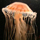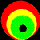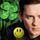## General Question# Is it possible to for an equation to equal anything depending on one variable?

Asked by dotlin (422) July 17th, 2010

Am I right in thinking if we had any number of variables and 1 undefined variable and depending on what that one undefined variable is the equation could equal anything.

E.G n: undefined x: could be 4 y: could be 7 x/y+n=what ever value we want depending on what n is even an irrational number.

Observing members: 0Composing members: 0Yes.

theichibun (2237)“Great Answer” (0) Flag as…@theichibun
Are there any proofs to show so?

The answer seems so obvious but I just want to know there isn’t a case I’m missing out.

dotlin (422)“Great Answer” (0) Flag as…You’re looking at an equation with 2 variables. 1+x=y Figure out what you want y to be. There’s always a number you can add to 1 to get the the number you want for y.

theichibun (2237)“Great Answer” (0) Flag as…@theichibun
No any number of variables what you can define how you like, x could equal infinity or 0 we can have as many variables as we want in this expression.

so x: infinity

x+n=10 what would n have to be for the equation to equal 10?

dotlin (422)“Great Answer” (0) Flag as…No. Think of the definition of rational and irrational numbers. If x and y are rational then N cannot be irrational.

Another case might be y = x + (n-x). The unknown x has no effect. The answer is always y n.

LuckyGuy (38679)“Great Answer” (0) Flag as…I am trying to figure out what you are asking. If all the variables are known except for one then what you have is one unknown, and the question would be if an equation in one variable can take on any value. Then if you have 1/x=0, there would be no solution.

LostInParadise (28166)“Great Answer” (0) Flag as…Thought about this some more. If you are just looking at polynomials, the answer is yes. If you are looking at functions like x^2+ 1/(x-2) + x^3 + 1/(x+7), then this is a rational function that can be expressed as p(x)/q(x) where p and q are polynomials. Then to solve for a value of n, you would have p/q = n or p(x)=n q(x) or p(x) – n q(x), which is a polynomial and would be solvable for any n provided that n is not a root of q(x)

LostInParadise (28166)“Great Answer” (0) Flag as…First off, infinity isn’t a number.

Second, I don’t think you understand you’re question.

x/y+n=W
4/7+n=W

Go solve that. You can’t solve for n because you don’t know what W is. Once you establish W’s value then you can solve for n. And if you establish a value for W then W is no longer anything, it’s the value that you’ve established that it has.

theichibun (2237)“Great Answer” (0) Flag as…or